• 信号包络熵的仿真计算及特点分析，计算信号的包络谱熵值，提取最小包络熵
• 包络matlab代码自动高频振荡检测（AHFOD） 该代码仍在开发中，因此欢迎您提出建议，并且不作任何保证。 该代码旨在用于检测以下数据中的高频振荡（HFO）： 脑电图脑电图（未经测试） 皮质脑电图ECog（已测试） ...
• 针对从滚动轴承振动信号中所提取的故障信息精度低的问题，利用鲸鱼优化算法对变分模态分解模态个数K和惩罚参数α 寻优，然后根据VMD处理信号得到若干模态分量，筛选后进一步提取能量作为特征向量。使用特征提取...目录
1 简介
2 变分模态分解VMD原理
3 鲸鱼优化算法优化VMD原理
3.1. 鲸鱼优化算法优化VMD原理及流程
3.2. 特征提取流程
4 优化效果
4.1. VMD各分量信号时域图
4.2. 鲸鱼优化算法优化VMD各分量信号时域图
4.3 全部图像
5. 主要代码
main.m
woa_vmd.m

1 简介

针对从滚动轴承振动信号中所提取的故障信息精度低的问题，利用鲸鱼优化算法对变分模态分解模态个数K和惩罚参数α 寻优，然后根据VMD处理信号得到若干模态分量，筛选后进一步提取能量熵作为特征向量。使用特征提取向量，可进行后续轴承故障诊断等工作。

2 变分模态分解VMD原理

VMD能够自适应匹配每种模态的最佳中心频率和有限带宽，实现固有模态分量（IMF）有效分离， 其核心思想是构建和求解变分问题。

VMD实现步骤为：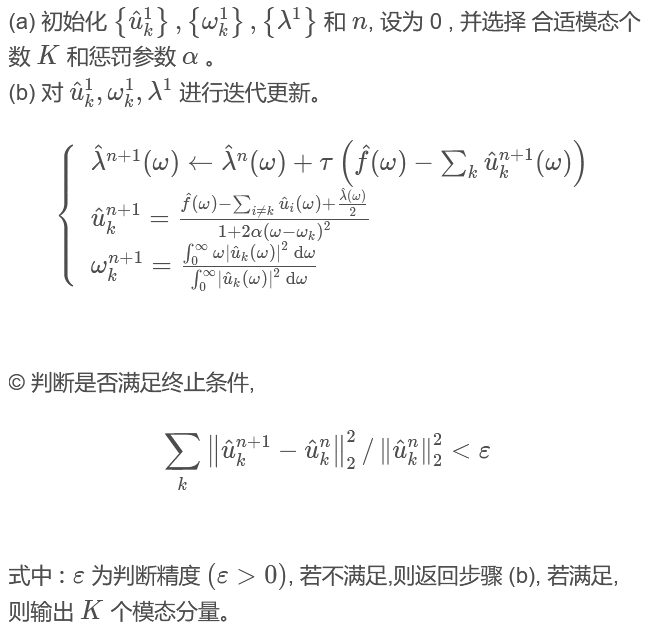由VMD分解步骤可知，分解信号前需要设置合适的模态个数K和惩罚参数α，K取值过大会导致过分解，反之，则会欠分解，α 取值过大，会造成频带信息丢失，反之，会信息冗余，所以需要确定最佳参数组合[K，α]。目前多用中心频率观察法，通过观察不同K值下的中心频率确定K值，但该法具有偶然性，且只能确定模态个数K，无法确定惩罚参数α。

3 鲸鱼优化算法优化VMD原理

3.1. 鲸鱼优化算法优化VMD原理及流程

使用鲸鱼优化算法（WOA）对VMD参数进行寻优，以包络熵极小值作为适应度函数，包络熵代表原始信号的稀疏特性，当IMF中噪声较多，特征信息较少时，则包络熵值较大，反之，则包络熵值较小。

信号x (i )(i = 1,2,…,N )包络熵EP 用以下公式计算，式中a (i ) 是由VMD 分解的k 个模态分量经Hilbert 解调后的包络信号，ε (i )是通过计算a (i )的归一化得到的概率分布序列，N 为采样点数，计算概率分布序列ε (i )的熵值即为包络熵EP。

采用WOA优化VMD参数流程如图所示。首先初始化鲸群位置向量[K，α]，以包络熵作为适应度函数，并计算每个鲸鱼适应度，然后通过判断收敛因子大小选择迭代公式进行迭代更新，直到满足终止条件，输出最优VMD参数。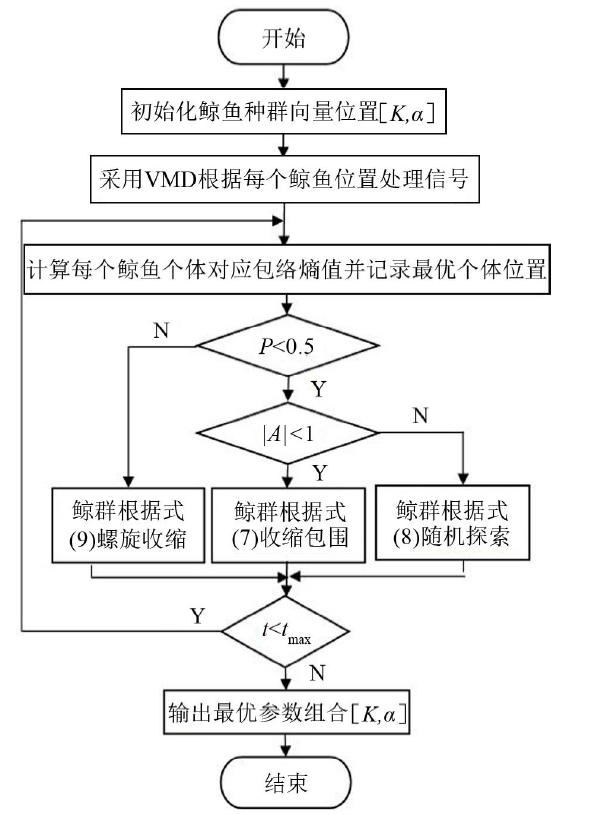3.2. 特征提取流程
VMD 利用WOA寻优后的参数组合[K,α]采用VMD对原信号分解，得到K个模态分量，计算每个IMF 峭度值，将其定义为：

式中：μ为信号x 的均值，σ 为信号x 的标准差。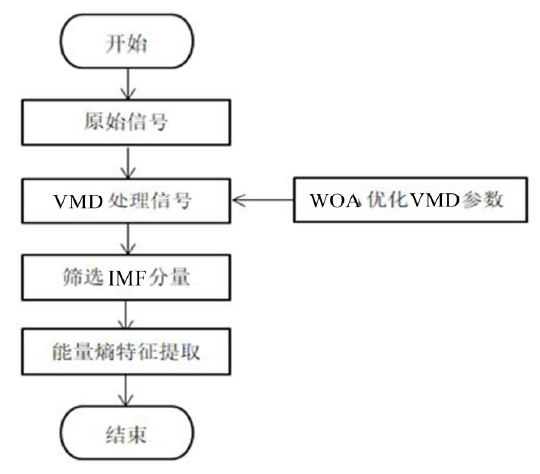4 优化效果

4.1. VMD各分量信号时域图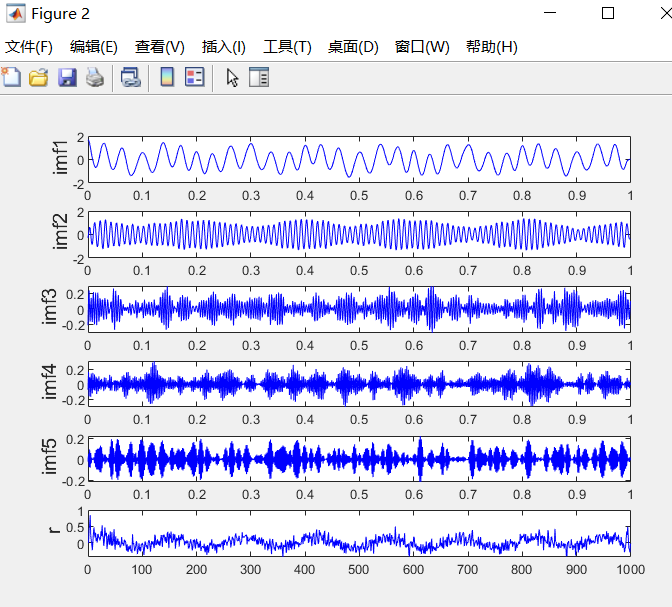4.2. 鲸鱼优化算法优化VMD各分量信号时域图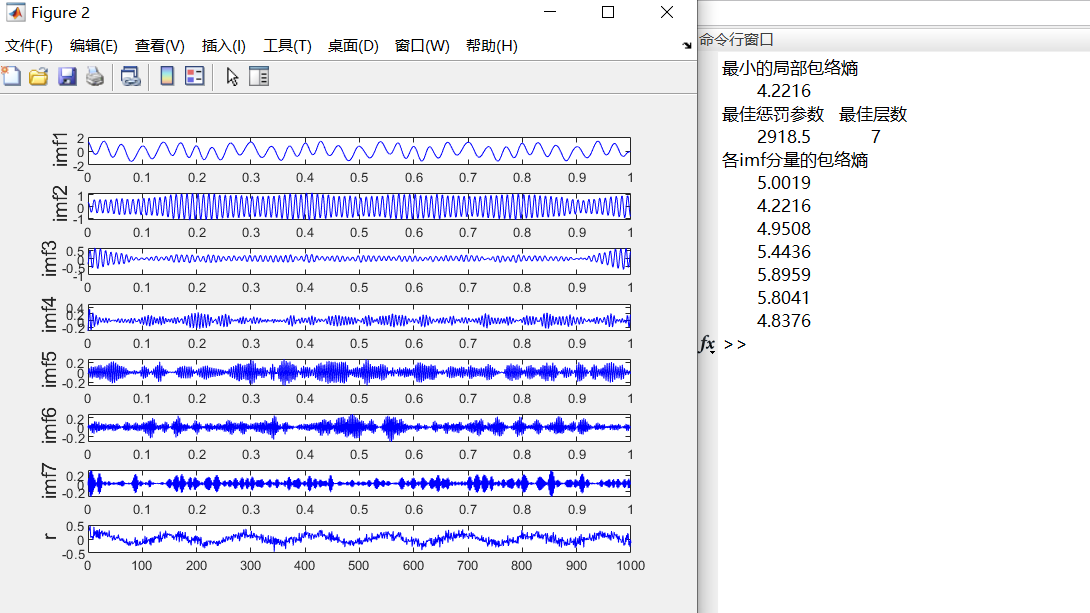4.3 全部图像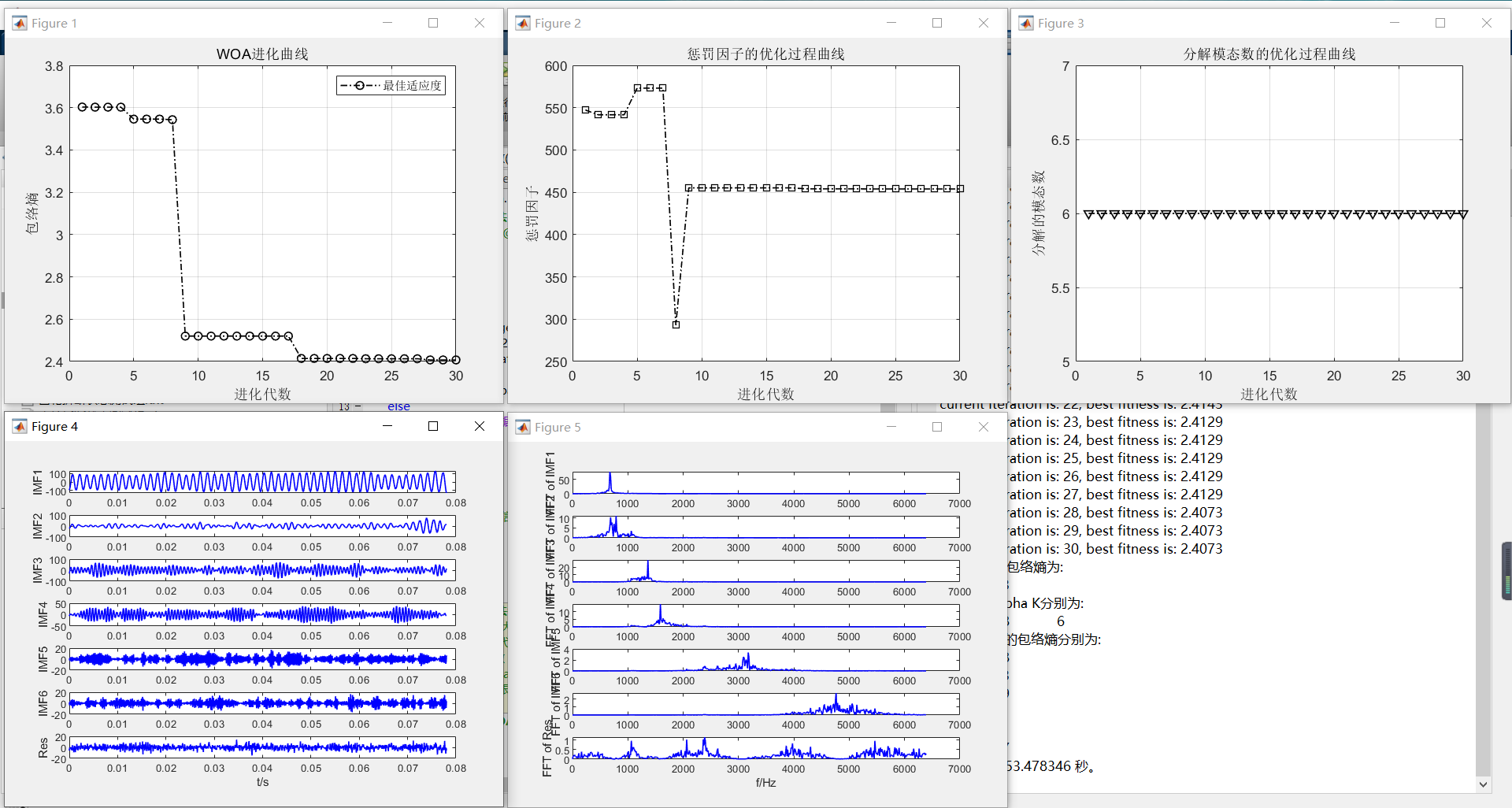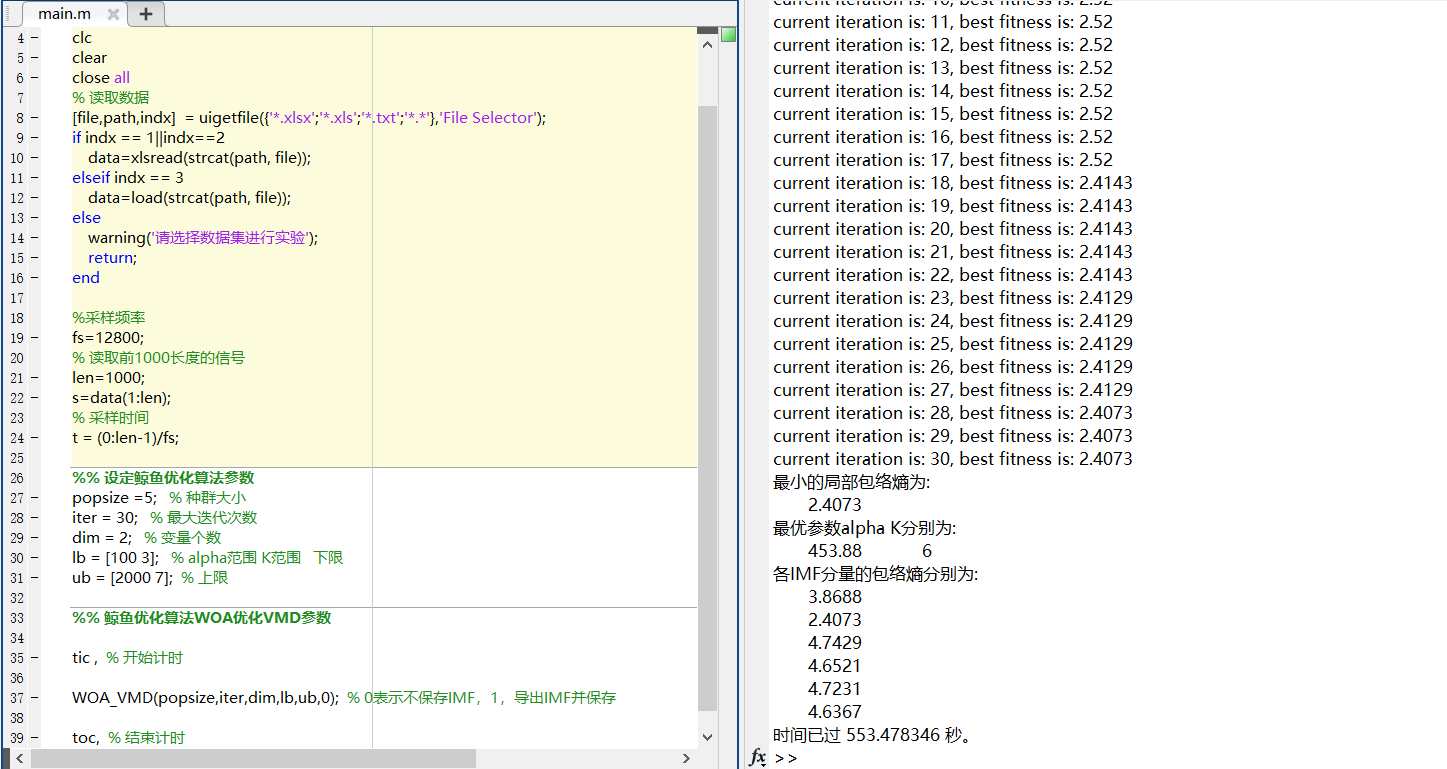5. 主要代码

main.m

%% 基于鲸鱼优化算法(whale optimization algorithm, WOA)优化变分模态分解（variational mode decomposition,VMD）参数

clc
clear
close all
warning off

% 读取数据
[file,path,indx]  = uigetfile({'*.xlsx';'*.xls';'*.txt';'*.*'},'File Selector');
if indx == 1||indx==2
elseif indx == 3
else
warning('请选择数据集进行实验');
return;
end

%采样频率
fs=12800;
% 读取前1000长度的信号
len=1000;
s=data(1:len);
% 采样时间
t = (0:len-1)/fs;

%% 设定鲸鱼优化算法参数
popsize =20;   % 种群大小
iter = 30;   % 最大迭代次数
dim = 2;   % 变量个数
lb = [100 3];   % alpha范围 K范围   下限
ub = [2000 7];  % 上限

%% 鲸鱼优化算法WOA优化VMD参数

tic ,  % 开始计时

WOA_VMD(popsize,iter,dim,lb,ub,0);  % 0表示不保存IMF，1，导出IMF并保存

toc,  % 结束计时


woa_vmd.m

function WOA_VMD(varargin)
% 子函数用于鲸鱼算法优化VMD的惩罚系数alpha和分解层数K

% initialize position vector and score for the leader，初始化位置向量和领导者得分
Leader_score=inf; %change this to -inf for maximization problems，将此更改为-inf以获得最大化问题，Inf无穷大

%Initialize the positions of search agents
Positions=initialization(SearchAgents_no,dim,ub,lb);%Positions，存放数个个体的多维位置。

Convergence_curve=zeros(1,Max_iteration);%Convergence_curve收敛曲线

gbest_array = zeros(Max_iteration,2);   % 初始化最优参数数组
% Main loop
for T=1:Max_iteration

for i=1:size(Positions,1)%对每个个体一个一个检查是否越界

% Return back the search agents that go beyond the boundaries of
% the search space，返回超出搜索空间边界的搜索代理
Flag4ub=Positions(i,:)>ub;
Flag4lb=Positions(i,:)<lb;
Positions(i,:)=(Positions(i,:).*(~(Flag4ub+Flag4lb)))+ub.*Flag4ub+lb.*Flag4lb;%超过最大值的设置成最大值，超过最小值的设置成最小值

% Calculate objective function for each search agent，目标函数值的计算
fitness=fun(Positions(i,:),s);

if fitness<Leader_score % Change this to > for maximization problem
end

end

disp(['current iteration is: ',num2str(T), ', best fitness is: ', num2str(Leader_score)])
a=2-T*((2)/Max_iteration); % a decreases linearly fron 2 to 0 in Eq. (2.3)

% a2 linearly dicreases from -1 to -2 to calculate t in Eq. (3.12)
a2=-1+T*((-1)/Max_iteration);

% Update the Position of search agents，参数更新
for i=1:size(Positions,1)
r1=rand(); % r1 is a random number in [0,1]
r2=rand(); % r2 is a random number in [0,1]

A=2*a*r1-a;  % Eq. (2.3) in the paper
C=2*r2;      % Eq. (2.4) in the paper

b=1;               %  parameters in Eq. (2.5)
l=(a2-1)*rand+1;   %  parameters in Eq. (2.5)

p = rand();        % p in Eq. (2.6)

for j=1:size(Positions,2)%对每一个个体地多维度进行循环运算

if p<0.5%收缩包围机制
if abs(A)>=1
D_X_rand=abs(C*X_rand(j)-Positions(i,j)); % Eq. (2.7)
Positions(i,j)=X_rand(j)-A*D_X_rand;      % Eq. (2.8)

elseif abs(A)<1
end

elseif p>=0.5%螺旋更新位置

% Eq. (2.5)

end

end
end

end
参考文献：张萍,张文海,赵新贺,吴显腾,刘宁.WOA-VMD算法在轴承故障诊断中的应用[J].噪声与振动控制,2021,41(04):86-93+275.

展开全文matlab 算法
• 包络matlab代码自动高频振荡检测（AHFOD） 该代码仍在开发中，因此欢迎您提出建议。 该代码旨在用于检测以下数据中的高频振荡（HFO）： 脑电图脑电图（未经测试） 皮层脑电图ECoG（已测试） 颅内脑电图iEEG（已...
• 针对从滚动轴承振动信号中所提取的故障信息精度低的问题，利用遗传算法对变分模态分解模态个数K和惩罚参数α 寻优，目标函数为多尺度排列参数，再根据VMD处理信号得到若干模态分量，筛选后进一步提取能量作为...目录
1 简介
2 变分模态分解VMD原理
3 GA优化VMD效果
3.1 遗传算法优化VMD各分量信号图像
3.2 全部图像
4 主要代码
main.m
GA_VMD.m

1 简介

针对从滚动轴承振动信号中所提取的故障信息精度低的问题，利用遗传算法对变分模态分解模态个数K和惩罚参数α 寻优，目标函数为多尺度排列熵参数，再根据VMD处理信号得到若干模态分量，筛选后进一步提取能量熵作为特征向量。使用特征提取向量，可进行后续轴承故障诊断等工作。

2 变分模态分解VMD原理

VMD能够自适应匹配每种模态的最佳中心频率和有限带宽，实现固有模态分量（IMF）有效分离， 其核心思想是构建和求解变分问题。

VMD实现步骤为：由VMD分解步骤可知，分解信号前需要设置合适的模态个数K和惩罚参数α，K取值过大会导致过分解，反之，则会欠分解，α 取值过大，会造成频带信息丢失，反之，会信息冗余，所以需要确定最佳参数组合[K，α]。目前多用中心频率观察法，通过观察不同K值下的中心频率确定K值，但该法具有偶然性，且只能确定模态个数K，无法确定惩罚参数α。

使用遗传算法（GA）对VMD变分模态分解的惩罚参数alpha与分解模态层数K进行寻优，以局部包络熵极小值作为适应度函数。[alpha, K] 为进化个体的位置，局部包络熵极小值为个体的适应度值。

3 GA优化VMD效果

3.1 遗传算法优化VMD各分量信号图像

1.时域图像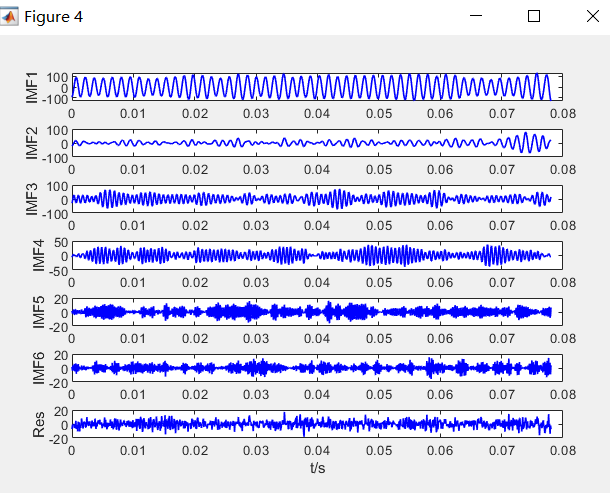2.频谱图像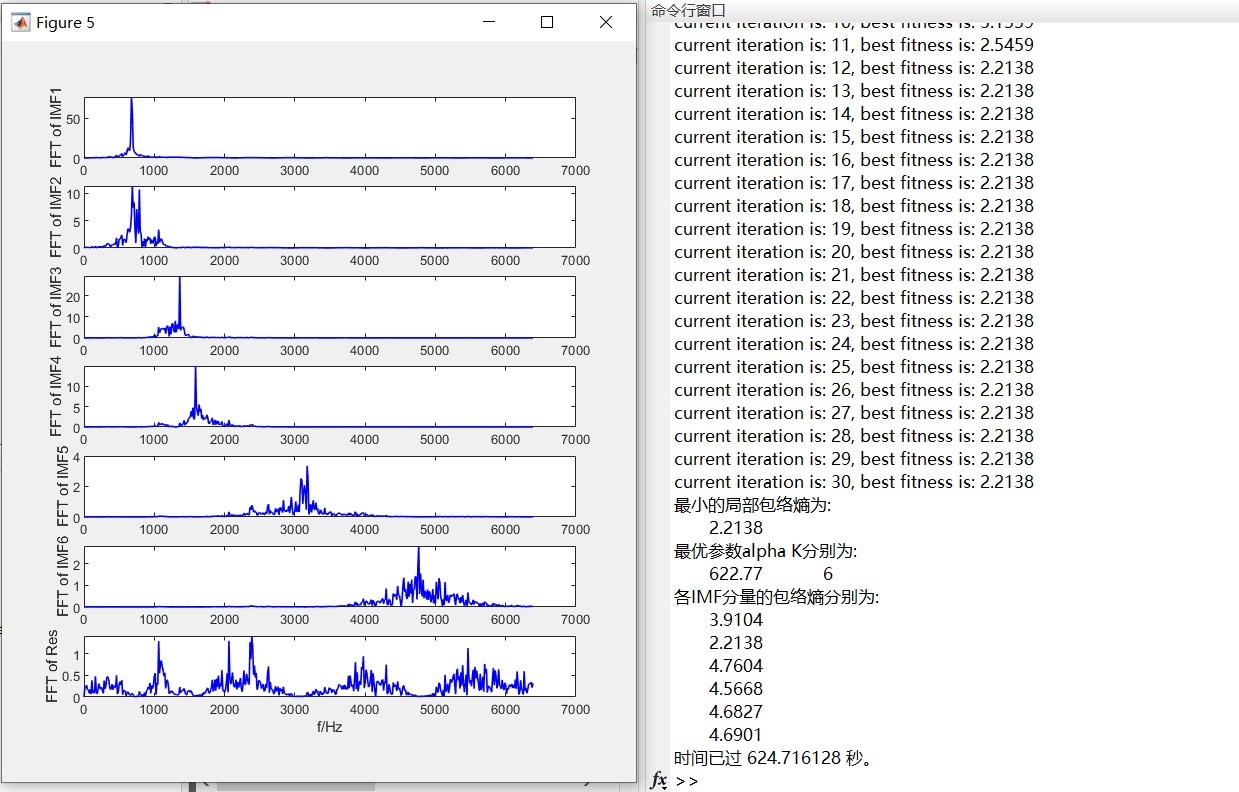3.2 全部图像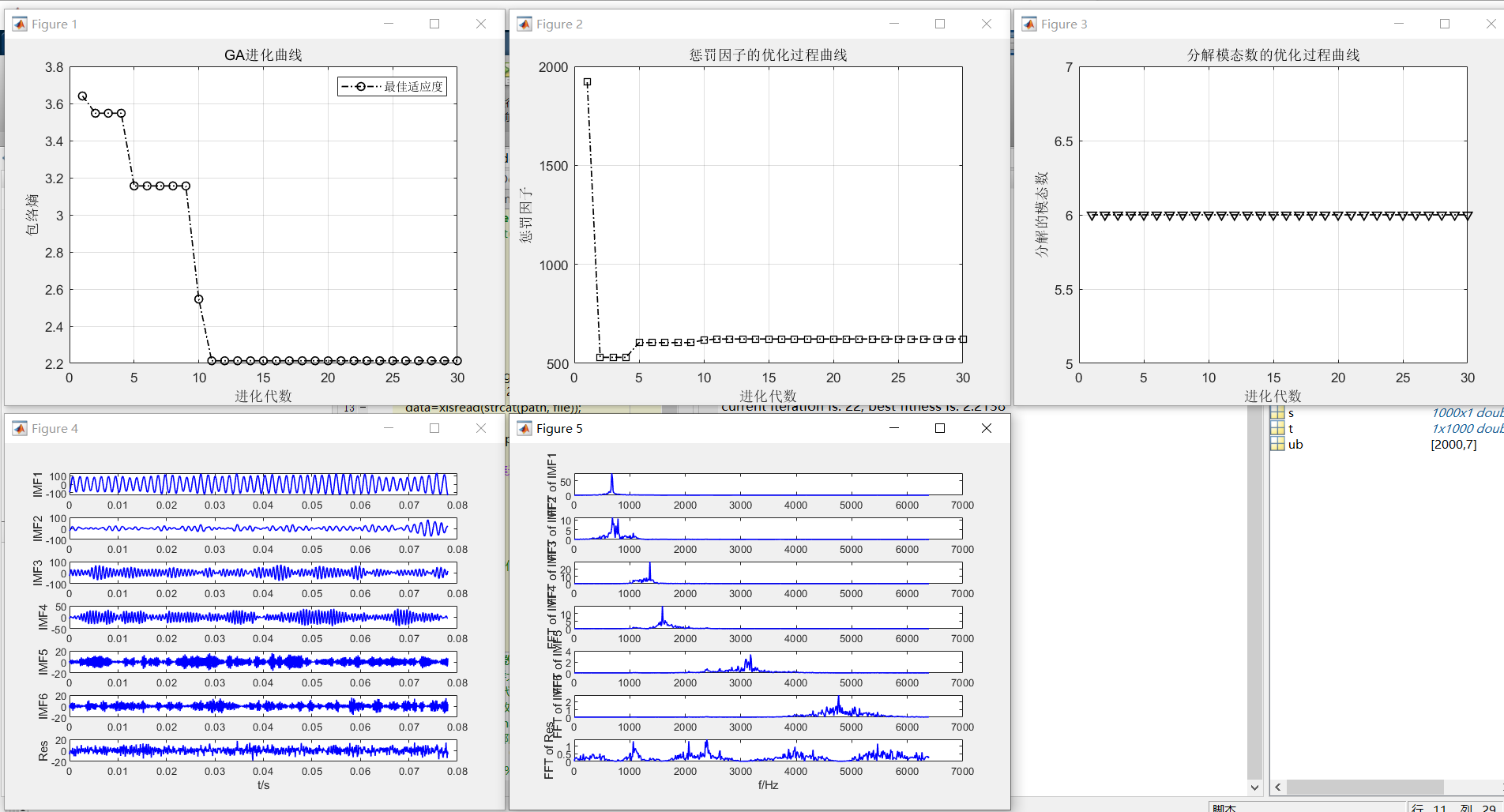4 主要代码

main.m

%% 基于遗传算法(genetic algorithm, GA))优化变分模态分解（variational mode decomposition,VMD）参数

clc
clear
close all
% 读取数据
[file,path,indx]  = uigetfile({'*.xlsx';'*.xls';'*.txt';'*.*'},'File Selector');
if indx == 1||indx==2
elseif indx == 3
else
warning('请选择数据集进行实验');
return;
end

%采样频率
fs=12800;
% 读取前1000长度的信号
len=1000;
s=data(1:len);
% 采样时间
t = (0:len-1)/fs;

%% 设定遗传算法参数
popsize =6;   % 种群大小
iter =30;   % 最大迭代次数
dim = 2;   % 变量个数
lb = [100 3];   % alpha范围 K范围   下限
ub = [2000 7];  % 上限
pc=0.8;                       %交叉概率，0和1之间
pm=0.2;                    %变异概率，0和1之间

%% 遗传算法GA优化VMD参数

tic ,  % 开始计时

GA_VMD(popsize,iter,dim,lb,ub,pc,pm,0);  % 0表示不保存IMF，1，导出IMF并保存

toc,  % 结束计时


GA_VMD.m

% 初始化GA的参数信息
lenchrom=ones(1,dim);       %每个变量的字串长度，如果是浮点变量，则长度都为1
lb=lb.*ones(1,dim);
ub=ub.*ones(1,dim);
bound=[lb;ub]';
% 初始化个体数组
individuals=struct('fitness',zeros(1,SearchAgents_no), 'chrom',[]);  %将种群信息定义为一个结构体
avgfitness=[];                      %每一代种群的平均适应度

%% 初始化种群计算适应度值
% 初始化种群
for i=1:SearchAgents_no
%随机产生一个种群
individuals.chrom(i,:)=Code(lenchrom,bound);
x=individuals.chrom(i,:);
%计算适应度
individuals.fitness(i)=fun(x,s);   %染色体的适应度
end

%找最好的染色体
[bestfitness, bestindex]=min(individuals.fitness);
bestchrom=individuals.chrom(bestindex,:);  %最好的染色体
avgfitness=sum(individuals.fitness)/SearchAgents_no; %染色体的平均适应度
% 记录每一代进化中最好的适应度
Convergence_curve=zeros(Max_iteration,1);
gbest_array=zeros(Max_iteration,2);
%% 迭代寻优
for i=1:Max_iteration
disp(['current iteration is: ',num2str(i), ', best fitness is: ', num2str(bestfitness)])
% 选择
individuals=select(individuals,SearchAgents_no);
avgfitness=sum(individuals.fitness)/SearchAgents_no;
%交叉
individuals.chrom=Cross(pc,lenchrom,individuals.chrom,SearchAgents_no,bound);
% 变异
individuals.chrom=Mutation(pm,lenchrom,individuals.chrom,SearchAgents_no,[i Max_iteration],bound);

% 计算适应度
for j=1:SearchAgents_no
x=individuals.chrom(j,:); %解码
individuals.fitness(j)=fun(x,s);
end

%找到最小和最大适应度的染色体及它们在种群中的位置
[newbestfitness,newbestindex]=min(individuals.fitness);
[~,worestindex]=max(individuals.fitness);
% 代替上一次进化中最好的染色体
if bestfitness>newbestfitness
bestfitness=newbestfitness;
bestchrom=individuals.chrom(newbestindex,:);
end
individuals.chrom(worestindex,:)=bestchrom;
individuals.fitness(worestindex)=bestfitness;

avgfitness=sum(individuals.fitness)/SearchAgents_no;

Convergence_curve(i,1)=bestfitness; %记录每一代进化中最好的适应度和平均适应度
gbest_array(i,:)=bestchrom;
end
%进化结束

展开全文matlab 算法
• 蛙跳算法优化VMD参数，惩罚系数，分解层数，matlab语言 ，最小包络熵为适应度函数。
蛙跳算法优化VMD参数，惩罚系数，分解层数，matlab语言 ，最小包络熵为适应度函数。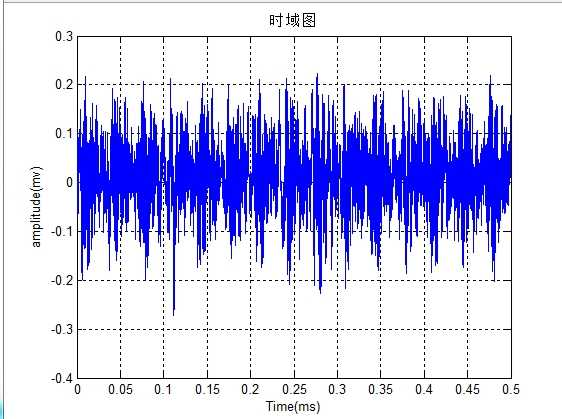展开全文• 振动信号中包络熵有什么作用，怎么用，有没有推荐的帖子，各位大神有没有相关学习材料matlab
• 针对普通工业采集风速，对其进行包络，hilbert。
• 包含常见的几种熵函数（样本，香农，样本，模糊）的MATLAB代码。
• 获取信号的数据文件来进行一系列处理，包括求信号的相关参数，做FFT，设计IIR滤波，画包络线，包络谱。
• 自己编写的包络分析程序，可以供大家学习交流 ，包络分析基本用法
• 基于DEAP数据集的特征提取———近似、排列、样本， 包含上述三个方法的python代码实现，全部在Jupyter Notebook上实现的。
• 利用经验模态分解提取IMF向量与残余向量的matlab程序，可以直接运行emdplus.m，亲测有效，不能运行不要钱~，主函数与样本熵函数samp经过了分析，里面有详细注解，EMD.m函数用的歪国人写的的~目前也是较为常用的版本...
• 该代码为MATLAB平台编写，可以计算一维图形包络信号处理
• 滚动轴承的包络谱分析；采用基于Hilbert包络解调的包络谱分析方法，能够清晰地显示与轴承故障特征频率成倍数关系的包络谱线，应用于轴承故障诊断
• ## 熵的计算

千次阅读 2018-01-23 16:11:28
def cal_entropy(dataset,m): #m表示计算dataset中第k列的 feature_cnt=Counter(dataset[:,m]) #引入Counter进行频度统计，或者采用字典的方式进行累加计算也可以； length=len(dataset) res= sum( [ -(v/...
#借用下python机器学习中的数据集

import numpy as np
from collections import Counter
from math import  log

def CreateDataSet():
dataset = np.array([[1, 1, 'yes' ],
[1, 1, 'yes' ],
[1, 0, 'no'],
[0, 1, 'no'],
[0, 1, 'no']])
return dataset

'''熵  h(x)  sum(-p(x)*log p(x) )'''
def cal_entropy(dataset,m): #m表示计算dataset中第k列的熵
feature_cnt=Counter(dataset[:,m])    #引入Counter进行频度统计，或者采用字典的方式进行累加计算也可以；
length=len(dataset)
res= sum( [ -(v/length)*log(v/length) for v in feature_cnt.values() ])
return res

datasets=CreateDataSet()  #这里label其实没什么用
print(cal_entropy(datasets,2))

'''联合熵，将两列特征拼接好后进行计算 h(x,y)  -p(x,y)*log p(x,y)'''

def cal_union_entropy(dataset,m,n):  #计算两个特征的联合熵
col1=dataset[:,m]
col2=dataset[:,n]
feature_cnt=Counter([str(col1[i])+col2[i] for i in range(len(col1))])
length=len(dataset)
res= sum( [ -(v/length)*log(v/length) for v in feature_cnt.values() ])
return res

print(cal_union_entropy(datasets,1,2))

'''条件熵   h(x,y)-h(x)  x确定时，y的不确定性 '''

def cal_condition_entropy(dataset,m,n):
return  cal_union_entropy(dataset,m,n)-cal_entropy(dataset,n)

print(cal_condition_entropy(datasets,1,2))

'''交叉熵 h(p,q)=sum(-p*log(q))'''

def cal_cross_entropy(dataset,m):
feature_cnt=Counter(dataset[:,m])    #引入Counter进行频度统计，或者采用字典的方式进行累加计算也可以；
length=len(dataset)
prob=[0.5,0.5]                  ###模拟一个q分布
value=list(feature_cnt.values())
res=sum([ - (value[i]/length)*log(prob[i]) for i in range(len(value)) ])
return res

print(cal_cross_entropy(datasets,2))

'''相对熵 kl(p||q)=sum(-p*log(q/p))'''

def cal_cross_entropy(dataset,m):
feature_cnt=Counter(dataset[:,m])    #引入Counter进行频度统计，或者采用字典的方式进行累加计算也可以；
length=len(dataset)
prob=[0.5,0.5]                  ###模拟一个q分布
value=list(feature_cnt.values())
res=sum([ - (value[i]/length)*log(prob[i]*length/value[i]) for i in range(len(value)) ])
return res

print(cal_cross_entropy(datasets,2))
分别输出：
0.6730116670092565 1.0549201679861442 0.38190850097688767 0.6931471805599453 0.020135513550688836
展开全文• 在网上看到一个博主写的有关样本的博客，由于该博客的博主已经停止更新博客，可能无法回答各位朋友的问题。所以本人在理解这篇博客的基础上，写了一篇有关样本的博客。对该样本程序做了修改，与原理公式更好...算法 matlab
• 文献为“基于 VMD 与 PSO 优化深度信念网络的 短期负荷预测”的caj文件，为提高短期负荷预测精度，采用变分模态分解(variational mode decomposition，VMD)技术将原始历史负荷序列分解为一系列特征互异的模态函数，...
• 今天在看局部方面的内容，看论文中介绍的内容感觉局部挺容易了，于是就有了实现的想法，结果效果非常糟糕。 得到的几乎是一张空白的图片，就像下面一样： 究其原因是各种论文上都写了这样一个公式： 这里...
• ## 样本熵的matlab程序

万次阅读 2017-12-15 11:36:19
转自http://www.ilovematlab.cn/thread-89427-1-1.html MATLAB下的动态样本计算 function SampEnVal = SampEn(data, m, r) %SAMPEN 计算时间序列data的样本 % data为输入数据序列 ...matlab EEG
• ## 谈谈“熵”

千次阅读 2014-12-22 21:45:22
（Entropy）在物理学中用于度量一个热力学系统的无序或混乱程度（参考热力学第二定律），当它被引入到信息论（计算机科学）时，则被用来衡量信息的不确定性（不可预测性），简单的说也就是“随机性”。 假设有...
• 利用VMD可以很好的分解信号，可以分解滚动轴承的原始数据和自己的信号仿真数据代码
• 信号处理； EMD；相关系数；Hilbert；包络谱 信号处理； EMD；相关系数；Hilbert；包络信号处理
• 可以清楚地看出信号的包络图，已经在matlab上成功运行，非常好用
• entropyEst.m-用于收敛的逐点估计 fm-归一化因子 filterLength.m-自适应滤波器窗口长度的更新 staticEstimationD.m-导数估计的初始化（将来可能会删除） staticEstimationW.m-包络估计的初始化（将来可能会删除） ...
• AM信号的波形包络和调制信号的包络一样，AM的信号的频谱包括载频分量，上边带和下边带。其中上边带的频谱结构和原调制信号的频谱结构一样，下边带是上边带的镜像。AM信号的总功率包括载波功率和边带功率。 31. 什么...
• ，谱，排列，小波，EMD包络等； 6、谱 峭度 ，快速谱峭度、小波谱峭度等； 7、基于数学工具和降维的特征，如 PCA，矩阵特征向量，矩阵的秩，特征根，SVD-奇异值、ICA 等等； 8、一些基于距离...matlab 信号特征 特征提取 时域
• 最小解卷积(Minimum Entropy Deconvolution,MED)通过最优滤波器对轴承微弱故障信号进行最优滤波,提高了信号的信噪比,然后对滤波后的信号进行包络解调分析,能够提取出信号中隐含的故障特征。将该方法应用于带式输送...
• 一、经验模态分解（Empirical Mode Decomposition, EMD） 目的 EMD是由 NE....它的优点是 不会运用任何已经定义好的函数作为基底 ...《基于 EEMD 和多尺度模糊的电机轴承故障特征提取方法研究 》EEMD
• 6、消息x所含的信息量的公式 单位比特bit，简称b P(x)表示消息发生的概率 I 表示消息中所含的信息量 P(x)越小，I越大 7、的公式： H(x)表示信息源的，简称，也表示平均信息量 当每个符号等概率独立出现时，......